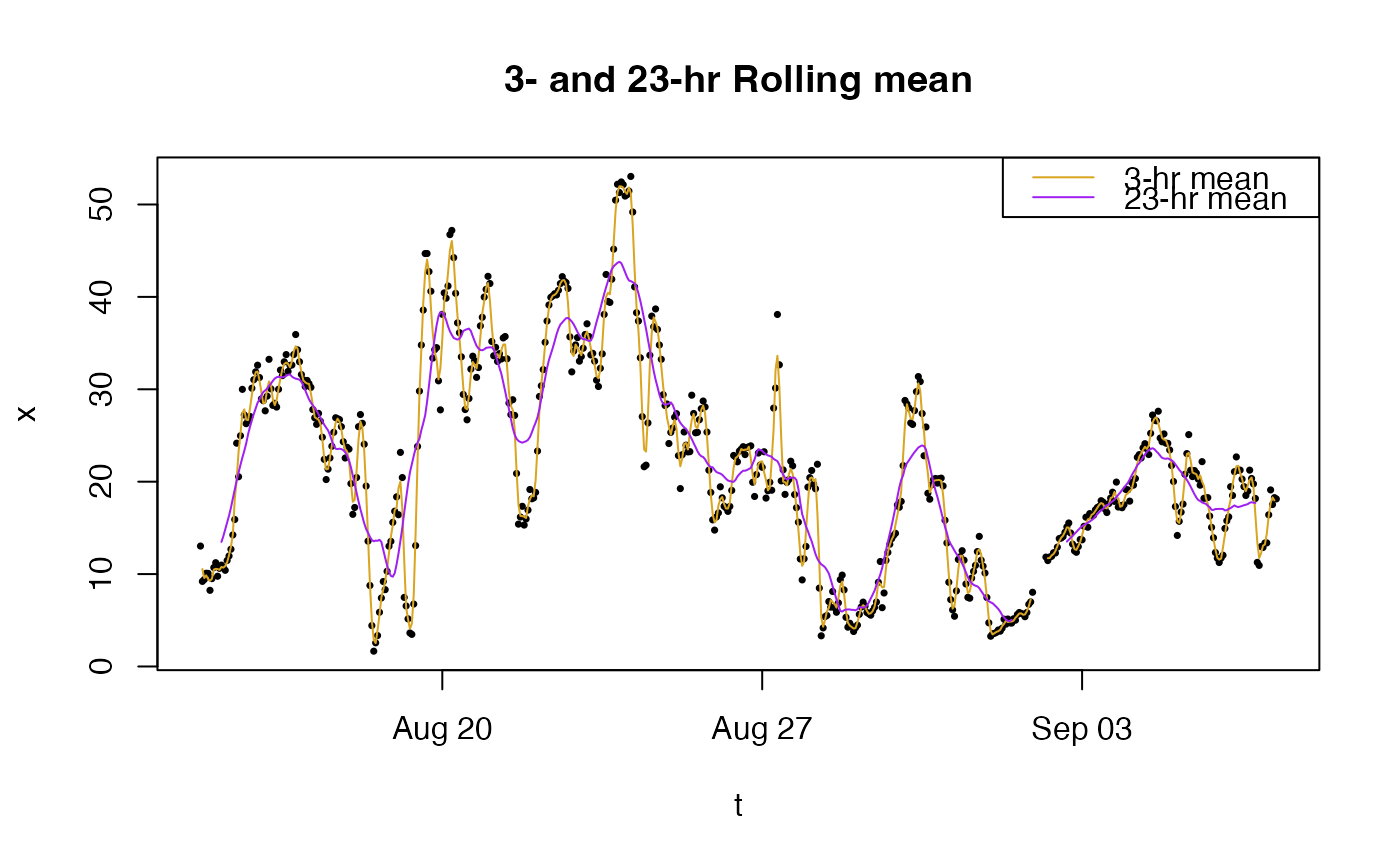Apply a moving-window mean function to a numeric vector.

roll_mean(
x,
width = 1L,
by = 1L,
align = c("center", "left", "right"),
na.rm = FALSE,
weights = NULL
)

## Arguments

x Numeric vector. Integer width of the rolling window. Integer shift by which the window is moved each iteration. Character position of the return value within the window. One of: "left" | "center" | "right". Logical specifying whether NA values should be removed before the calculations within each window. Numeric vector of size width specifying each window index weight. If NULL, unit weights are used.

## Value

Numeric vector of the same length as x.

## Details

For every index in the incoming vector x, a value is returned that is the mean of all values in x that fall within a window of width width.

The align parameter determines the alignment of the return value within the window. Thus:

• align = -1 [*------] will cause the returned vector to have width-1 NA values at the right end.

• align = 0 [---*---] will cause the returned vector to have width/2 NA values at either end.

• align = 1 [------*] will cause the returned vector to have width-1 NA values at the left end.

For large vectors, theby parameter can be used to force the window to jump ahead by indices for the next calculation. Indices that are skipped over will be assigned NA values so that the return vector still has the same length as the incoming vector. This can dramatically speed up calculations for high resolution time series data.

The roll_mean() function supports an additional weights argument that can be used to calculate a "weighted moving average" -- a convolution of the incoming data with the kernel (weighting function) provided in weights.

## Examples

library(MazamaRollUtils)

# Example air quality time series
t <- example_pm25$datetime x <- example_pm25$pm25

plot(t, x, pch = 16, cex = 0.5)
lines(t, roll_mean(x, width = 3), col = "goldenrod")
lines(t, roll_mean(x, width = 23), col = "purple")
legend("topright", lty = c(1, 1),
col = c("goldenrod", "purple"),
legend = c("3-hr mean", "12-hr mean"))
title("3- and 23-hr Rolling mean")